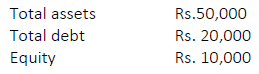Important Questions - Ratio (Financial Statement Analysis)

# Important Questions - Ratio (Financial Statement Analysis) - Accountancy Class 12 - Commerce

Important Questions

Q1. The current ratio of the company is 2.5:1. State giving reasons whether declaration of dividend will improve, reduce or not change the ratio.

(1)

Q2. If operating ratio of a company is 24% what is the operating profit ratio of the company?

(1)

Q3. Find debt equity and proprietary ratio and also comment upon the financial position of the business(3)

Q4. What will be the impact of the following transactions on debt equity ratio-.8:1
Sale of fixed assets at a profit
Declaration of dividend
Payment of dividend for dividend already declared

(3)

Q5. a) X Ltd. has a current ratio of 3.5:1 and quick ratio of 2:1. If excess of current assets over quick assets represented by Inventory is 24,000, calculate current assets and current liabilities.
b) From the following information, calculate Inventory Turnover Ratio.
Revenue from Operations: 4,00,000, Average Inventory : 55,000, The rate of Gross Loss on Revenue from Operations was 10%.

(4)

Q6. Net profit after Interest taxes is Rs2,80,000,15% long term debt Rs8,00,000. 10% preference Share capital Rs4,80,000, Tax rate 30% Calculate return on capital employed and interest coverage ratio.

(4)

Q7. A company’s Stock Turnover is 5 times. Stock at the end is Rs. 20,000 more than that at the beginning. Revenue from operations are Rs. 8,00,000. Rate of Gross Profit on cost 1/4; Current Liabilities Rs. 2,40,000. Acid Test Ratio 0.75. Calculate Current Ratio

(4)

Q8. From the following information, calculate the following ratios:

(4)

(i) Acid Test Ratio (ii) Debt Equity Ratio (iii) Working Capital Turnover Ratio (iv) operating ratio
Information:
Net RFO 3,00,000; Gross Profit 1,00,000; Total Current Assets 2,00,000; Closing Inventory 20,000; Prepaid Insurance 4,000; Total Current Liabilities 1,20,000; Share Capital 3,50,000; Reserve & Surplus 40,000; Preliminary Expenses 7,000; Fixed Assets 4,30,000, selling and distribution expenses Rs.4,000 & office and administrative expenses Rs.6,000.

Q9. Current ratio- 2,Quick ratio- 1.5,Working capital- rs.3,00,000 Closing stock is 3times more than the opening stock, Average age of inventory 73 days, Gross profit is 10% of revenue from operations, Cash revenue from operations is ¼ of credit revenue from operations, Average debtors-Rs.50000.Find Cl. stock, cost of revenue from operations and debtors velocity.

(6)

The document Important Questions - Ratio (Financial Statement Analysis) | Accountancy Class 12 - Commerce is a part of the Commerce Course Accountancy Class 12.
All you need of Commerce at this link: Commerce

## Accountancy Class 12

57 videos|105 docs|68 tests

## FAQs on Important Questions - Ratio (Financial Statement Analysis) - Accountancy Class 12 - Commerce

 1. What is ratio analysis in financial statement analysis?Ans. Ratio analysis is a method used in financial statement analysis to evaluate the performance and financial health of a company. It involves calculating and interpreting various ratios, such as liquidity ratios, profitability ratios, and solvency ratios, to assess the company's ability to meet its financial obligations and generate profits.
 2. How is liquidity ratio calculated and what does it indicate?Ans. Liquidity ratio is calculated by dividing a company's current assets by its current liabilities. It indicates the company's ability to meet its short-term obligations using its current assets. A higher liquidity ratio suggests that the company has enough liquid assets to cover its short-term debts, while a lower ratio may indicate potential liquidity issues.
 3. What are profitability ratios and how are they useful in financial statement analysis?Ans. Profitability ratios measure a company's ability to generate profits from its operations. Some commonly used profitability ratios include gross profit margin, net profit margin, and return on assets (ROA). These ratios help investors and analysts assess the company's efficiency, profitability, and overall financial performance.
 4. How can solvency ratios help in assessing a company's financial stability?Ans. Solvency ratios are used to evaluate a company's long-term financial stability and its ability to meet its long-term obligations. Examples of solvency ratios include debt-to-equity ratio and interest coverage ratio. These ratios provide insights into a company's debt levels, leverage, and ability to service its debt, which are crucial factors in determining its financial health.
 5. What are the limitations of ratio analysis in financial statement analysis?Ans. While ratio analysis is a valuable tool, it has its limitations. Some common limitations include the reliance on historical data, difficulty in comparing ratios across different industries, and the influence of non-financial factors on a company's performance. Additionally, ratio analysis only provides a snapshot of a company's financial status and should be used in conjunction with other qualitative and quantitative analysis methods for a comprehensive assessment.

## Accountancy Class 12

57 videos|105 docs|68 testsExplore Courses for Commerce examSignup to see your scores go up within 7 days! Learn & Practice with 1000+ FREE Notes, Videos & Tests.
10M+ students study on EduRev
Track your progress, build streaks, highlight & save important lessons and more!
Related Searches

,

,

,

,

,

,

,

,

,

,

,

,

,

,

,

,

,

,

,

,

,

;